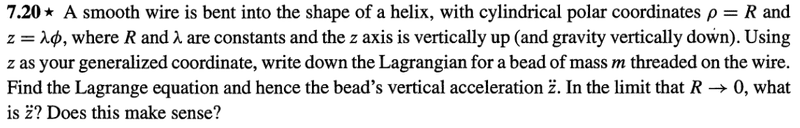# Bead on a Helix, angular velocity

## Homework Statement## Homework Equations

$$\mathcal{L}=T-U$$
$$\omega= \frac{d\phi}{dt}$$
$$I=mr^{2}$$

## The Attempt at a Solution

My problem is not finding the Lagrangian. But finding the kinetic energy! The translational kinetic energy would obviously be the following:

$$K.E t=\frac{1}{2}m(\dot{x}^{2}+\dot{y}^{2}+\dot{z}^{2})=\frac{1}{2}m(1+(\frac{R}{\lambda})^{2}) \dot{z}^{2}$$

But as far as I understood, there is rotational kinetic energy as well, namely the following

$$K.E r= \frac{1}{2}I \omega^{2} = \frac{1}{2}mR^{2} \frac{\dot{z}^2}{\lambda^2}$$

The helix is just the trajectory of the particle. If you look at the helix from above you see a circle. Furthermore, the angle depend on ##z##, which I believe support my idea.

I usually prefer looking at the solutions. Especially when I self study. I looked at two different sources from two different universities, none include rotational kinetic energy! Am I wrong?

Source 1
Source 2

Footnote: Classical Mechanics, By Taylor. Problem 7.20

#### Attachments

BvU
Homework Helper
Hi Phy,

Straight answer is yes. You are double counting the motion in the x-y plane. ##{1\over 2}I\omega^2## is the kinetic energy of a point mass moving in a circle.
If the bead would be of finite size, there would be an extra kinetic energy term from rotation of the bead around its ##z##-axis.

Hi Phy,

Straight answer is yes. You are double counting the motion in the x-y plane. ##{1\over 2}I\omega^2## is the kinetic energy of a point mass moving in a circle.
If the bead would be of finite size, there would be an extra kinetic energy term from rotation of the bead around its ##z##-axis.

So, unless an object has a shape, translational kinetic energy is enough to describe kinetic energy as whole.
Once an object has a shape, and its changing direction in general (in a plane or volume/or rotating around an axis), translational kinetic energy is not enough.

What I stated is correct?

BvU
Homework Helper
Check out how the expression ##{1\over 2} I \omega^2## is derived in a suitable textbook: it is simply the integral of the kinetic energies of the constituents of an extended body.
What you state is correct -- I think. If it helps understanding seems a bit doubtful to me.

•Phylosopher
vela
Staff Emeritus
Homework Helper
Once an object has a shape, and its changing direction in general (in a plane or volume/or rotating around an axis), translational kinetic energy is not enough.

What I stated is correct?
You have the right idea, but the distinction you're making between rotational and translational is not so clearcut.

Consider a point mass ##m## rotating about a point at a distance ##r## with angular speed ##\omega##. On the one hand, you could say the speed of the mass is ##v = \omega r##, so its translational kinetic energy is ##\frac 12 m (\omega r)^2##. On the other hand, you could say it has a rotational kinetic energy ##\frac 12 I\omega^2## where ##I = mr^2##. Either way, you get the same result for the kinetic energy. It depends on the way you want to look at the system.

Now consider a uniform rod of mass ##m## and length ##L## rotating with angular speed ##\omega## about an axis that passes through the end of the rod and is perpendicular to the rod. The moment of inertia of the rod about this axis is ##I = \frac 13 mL^2##, and its kinetic energy is
$$K = \frac 12 I \omega^2 = \frac 16 mL^2\omega^2.$$ We could also express its kinetic energy in terms of the motion of the center of mass and rotation about the center of mass. The center of mass of the rod moves with speed ##v_\text{cm} = \omega L/2##, and the moment of inertia about the center of mass is ##I_\text{cm} = \frac{1}{12}mL^2##. So the kinetic energy of the rod is
$$K = \frac 12 I_\text{cm} \omega^2 + \frac 12 m v_\text{cm}^2 = \frac 12\left(\frac {1}{12}mL^2\right)\omega^2 + \frac 12 m \left(\frac{\omega L}{2}\right)^2 = \frac 16 mL^2 \omega^2.$$ Finally, we could calculate the translational kinetic energy of each piece of the rod and integrate:
$$dK = \frac 12 v^2\,dm = \frac 12 v^2 \lambda\,dr \quad \Rightarrow \quad K = \frac 12 \int_0^L (\omega r)^2\ \lambda\,dr = \frac 16 mL^2 \omega^2,$$ where ##\lambda = m/L##.

•Phylosopher